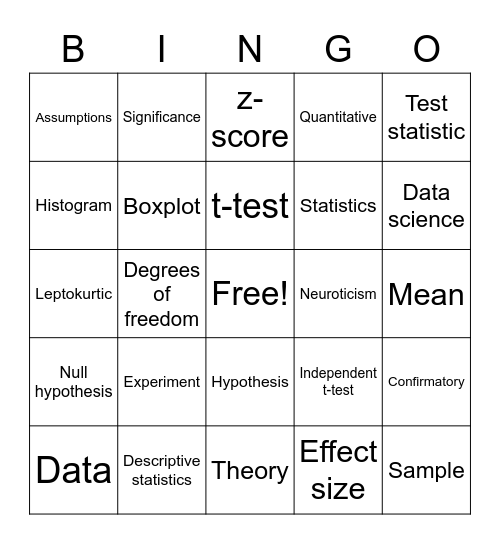# Statistics BingoThis bingo card has a free space and 49 words: Population, Sample, Central limit theorem, Theory, Experiment, Alternative hypothesis, Significance, p-value, Histogram, Misleading, Boxplot, Quantitative, Confirmatory, Independent t-test, Dependent design, One sample, t-test, Neuroticism, Extraversion, Test statistic, Degrees of freedom, Effect size, Assumptions, Statistics, Research, Data science, Data, .05, Hypothesis, Null hypothesis, Variables, Independent variable, Nominal variable, Interval variable, Descriptive statistics, Measures of central tendency, Inferential statistics, Median, Mean, Range, Histogram, Modality, Normal distribution, Negative skew, Leptokurtic, z-score, Type I error, Type II error and d.

⚠ This card has duplicate items: Histogram (2)

## Play Online